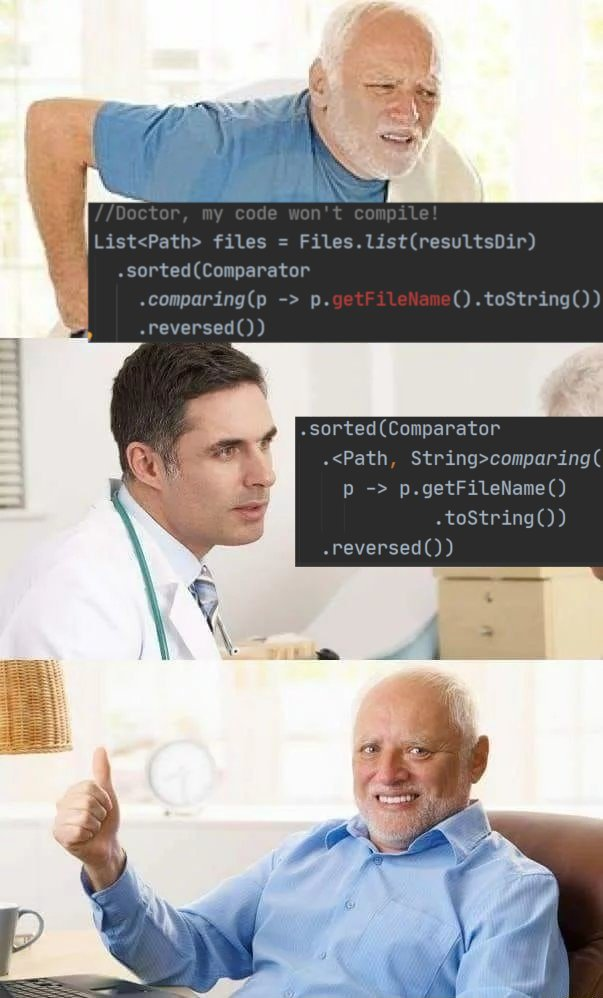# Core Java. Lecture #7

## Collections, lambdas, method references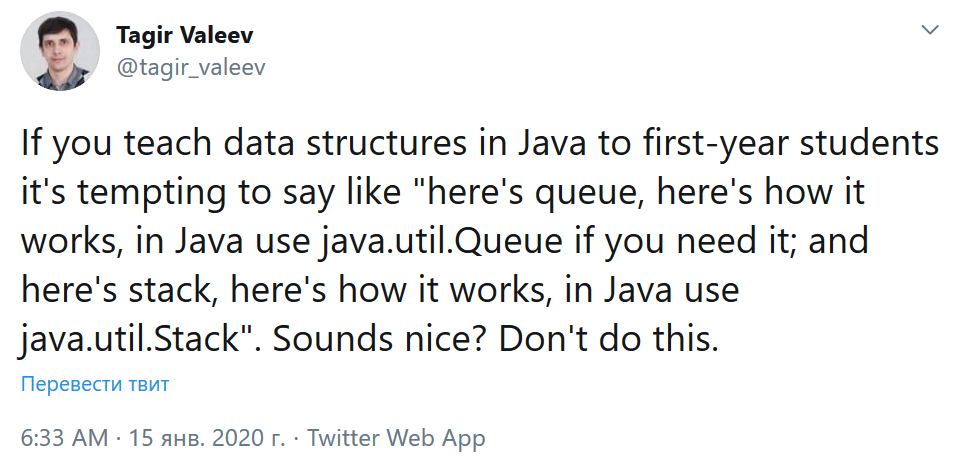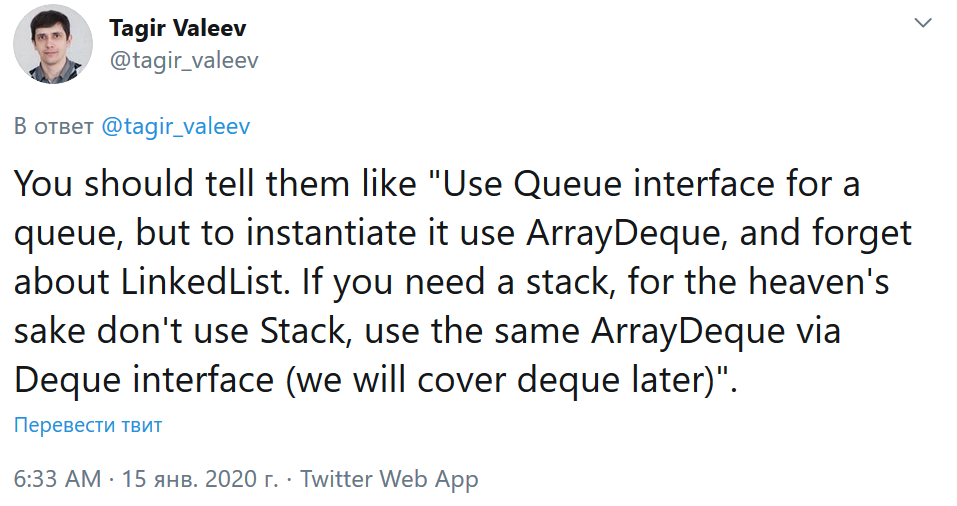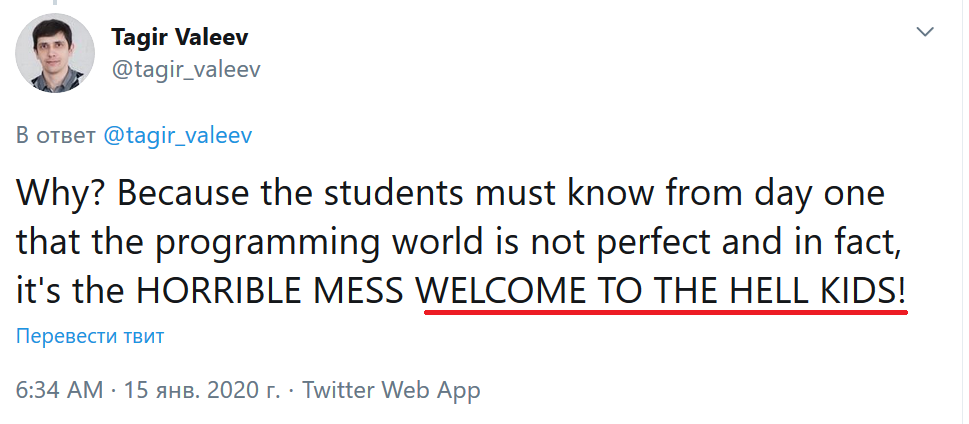## Collections: Separation of interfaces and implementations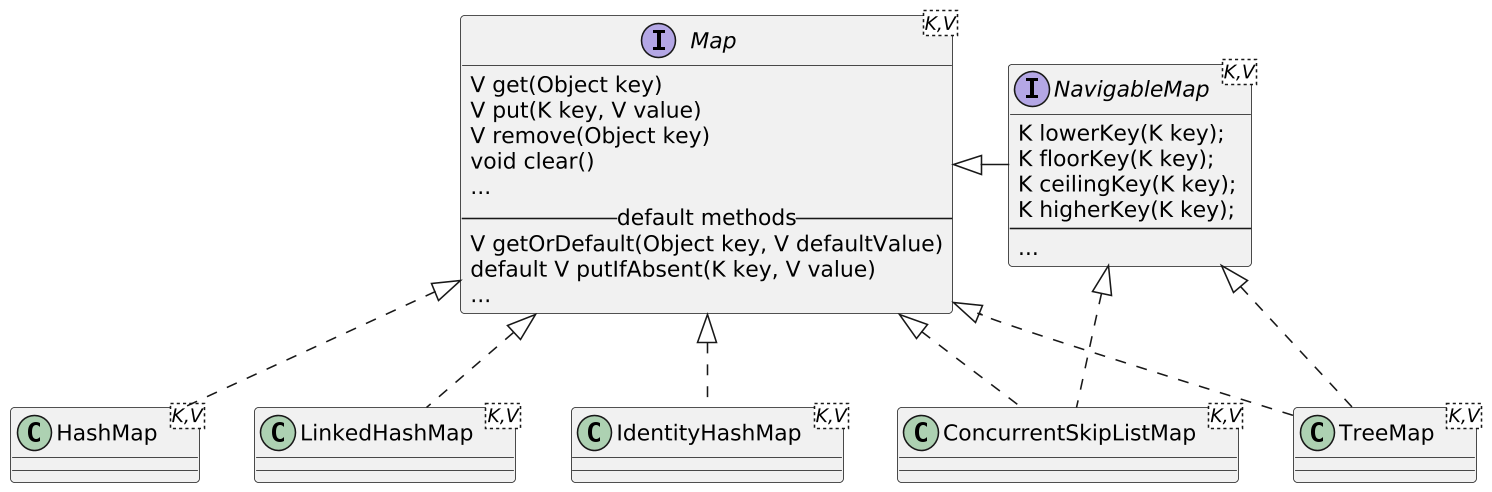## Collection Interfaces: Descendants of Collection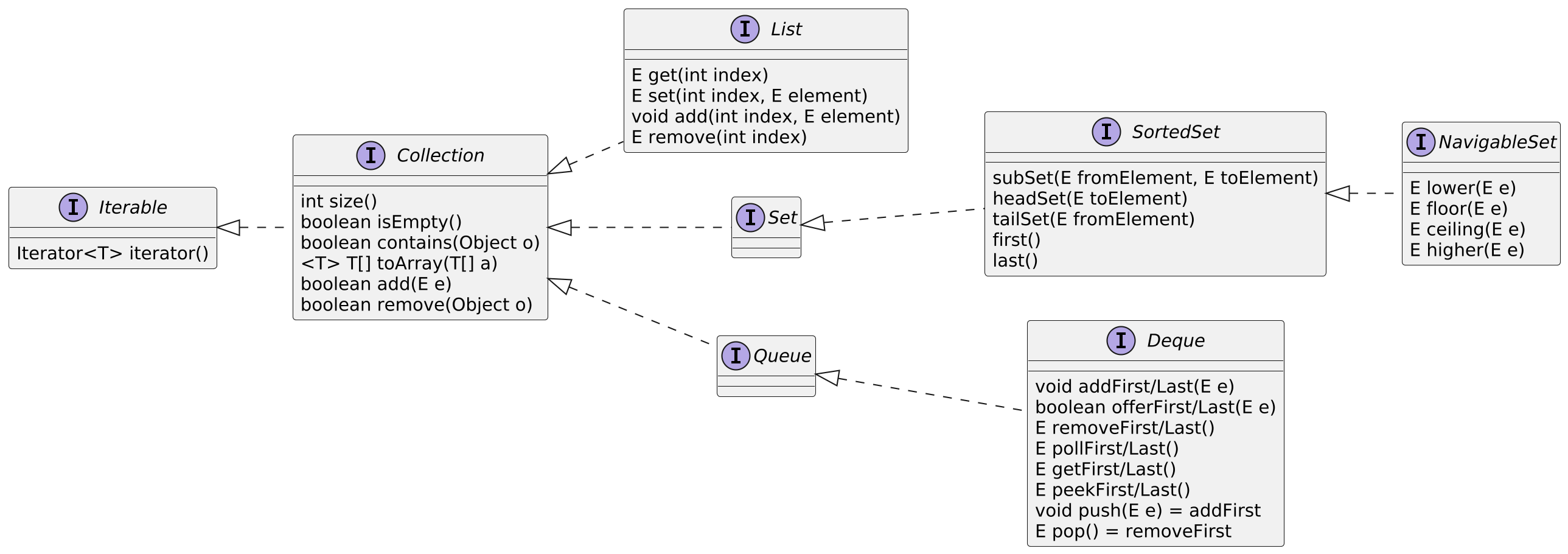## Iterable: an interface that can participate in `for` loop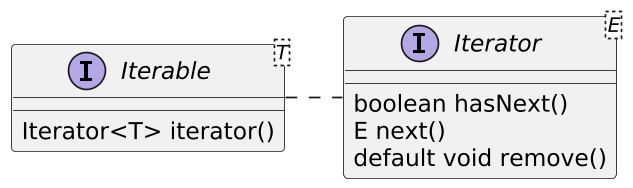``````Iterable collection = ... for (T e: collection) { ... }`````` ``````Iterable collection = ... Iterator i = collection.iterator(); while (i.hasNext()) { T e = i.next(); if (e...) i.remove(); }``````

## ListIterator: extension for List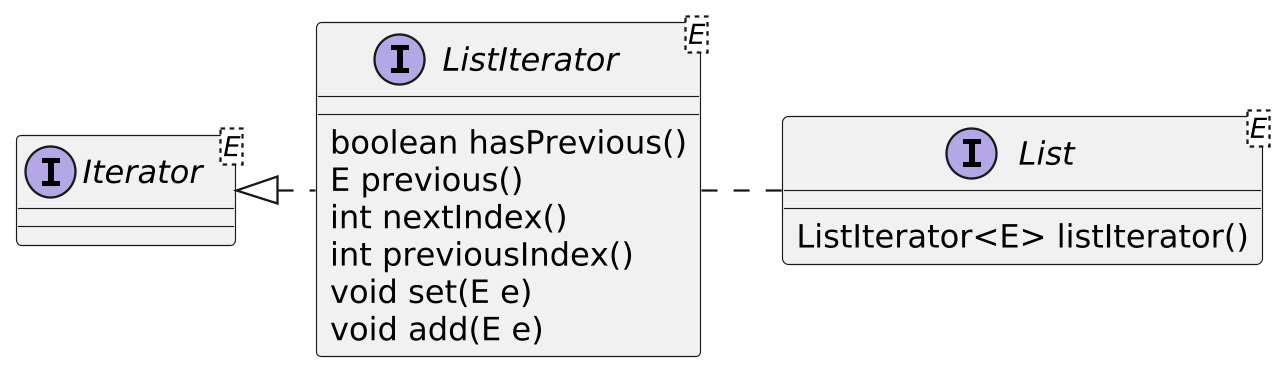## Collection Interfaces: Descendants of `Map`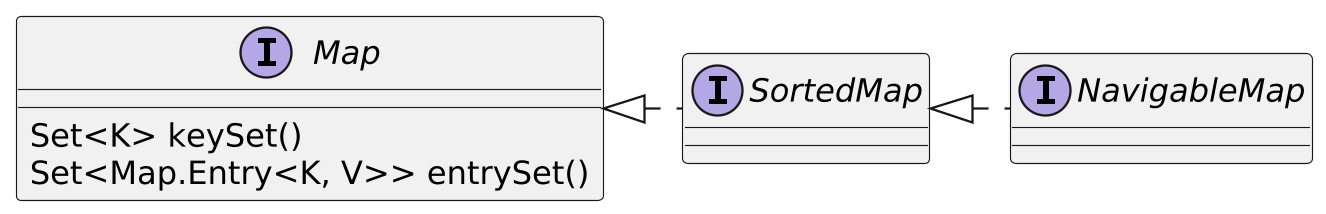## Comparable and Comparator

``````public interface Comparable<T>{
/**
* @param  o the object to be compared.
* @return a negative integer, zero, or a positive integer as this object
*         is less than, equal to, or greater than the specified object.
*/
int compareTo(T o);
}

/*Applies if objects being compared
do not implement Comparable*/
public interface Comparator<T> {
int compare(T o1, T o2);
}``````

## Intermediate Conclusions

• Use interfaces for variable types and method arguments. Don’t be tied to specific implementations.

• In addition to the possibility of substitution of implementations, there are surrogate collections, for example, the following important special cases:

``````//IMMUTABLE
//Empty                   //From a single element
Collections.emptyList();   Collections.singletonList(o);
Collections.emptySet();    Collections.singleton(o);
Collections.emptyMap();    Collections.singletonMap(k,v);``````

## Immutable collections of specified elements

``````//Of specified elements
List.of(a, b, c...);
Set.of(a, b, c...);
Map.of(k1, v1, k2, v2...);``````

But how did they do it for map?

## Surrogate collections: (partial) protection when an object is published

``````Collections.unmodifiableList(List<? extends T> l);
Collections.unmodifiableSet(Set<? extends T> s);
Collections.unmodifiableMap(Map<? extends K,
? extends V> s);
...``````

In the `Collections` class (as well as in the `Arrays` class) there is a lot of useful stuff!

## ArrayList the Great

``````List<String> list = new ArrayList<>();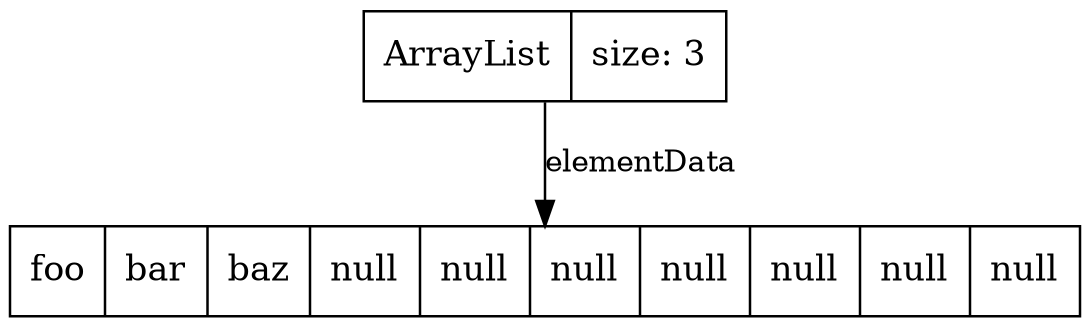(Vizualization is made with Lightweight Java Visualizer.)

## Algorithmic properties of `ArrayList`

• `get(int index)` is O(1)main benefit of `ArrayList<E>`

• `add(E element)` is O(1) amortized, but O(n) worst-case since the array must be resized and copied

• `add(int index, E element)` is O(n) (with n/2 steps on average)

• `remove(int index)` is O(n) (with n/2 steps on average)

• `Iterator.remove()` is O(n) (with n/2 steps on average)

• `ListIterator.add(E element)` is O(n) (with n/2 steps on average)

``````List<String> list = new LinkedList<>();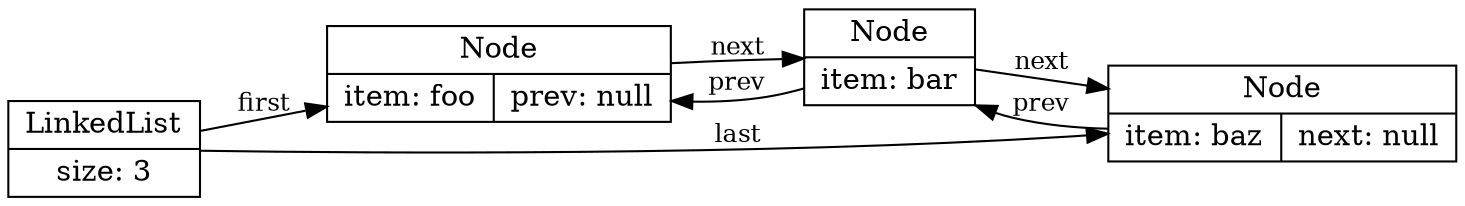## Algorithmic properties of `LinkedList`

• Implements `List` and `Deque`

• `get(int index)` is O(n) (with n/4 steps on average)

• `add(E element)` is O(1)

• `add(int index, E element)` is O(n) (with n/4 steps on average), but O(1) when `index = 0`main benefit of `LinkedList<E>`

• `remove(int index)` is O(n) (with n/4 steps on average)

• `Iterator.remove()` is O(1)main benefit of `LinkedList<E>`

• `ListIterator.add(E element)` is O(1) This is one of the main benefits of `LinkedList<E>`## What if you still need `Deque`?

• `ArrayDeque`

• Circular array

• Faster than `LinkedList`.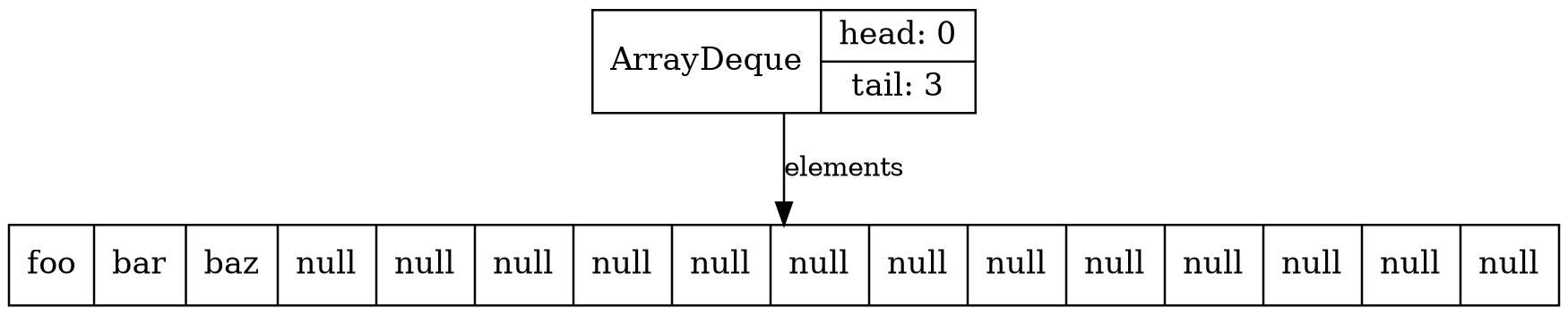## PriorityQueue

• Queuing by priority utilizing `Comparable` or `Comparator`.

• Balanced binary heap: "the two children of `queue[n]` are `queue[2*n+1]` and `queue[2*n+2]` "

``````PriorityQueue<String> q = new PriorityQueue<>();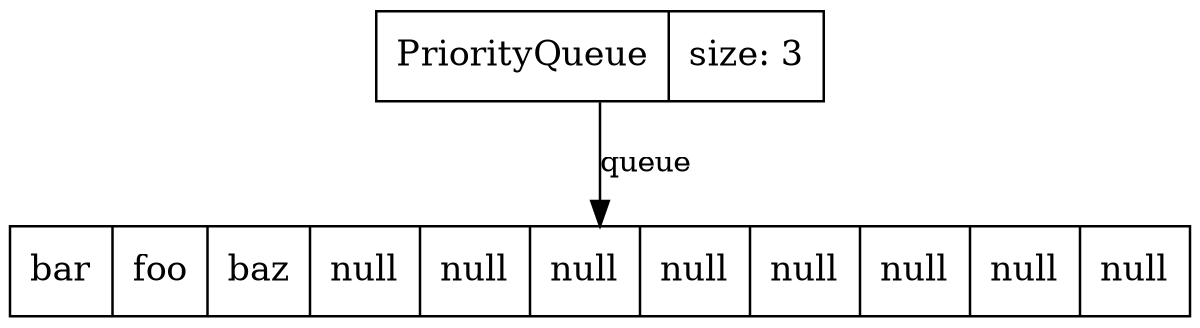• Theoretical asymptotics does not fully describe the suitability of a particular data structure: a number of circumstances should be taken into account, such as

• particular "sweet spot" cases and their frequency,

• cache usage efficiency,

• amount of "garbage" produced

• etc.

• `ArrayList` and `ArrayDeque` are the preferred choice as implementation of `List` and `Deque` in most of the cases.

## HashMap the Great

``````Map<String, Integer> map = new HashMap<>();
map.put("foo", 1); map.put("bar", 2); map.put("baz", 3);``````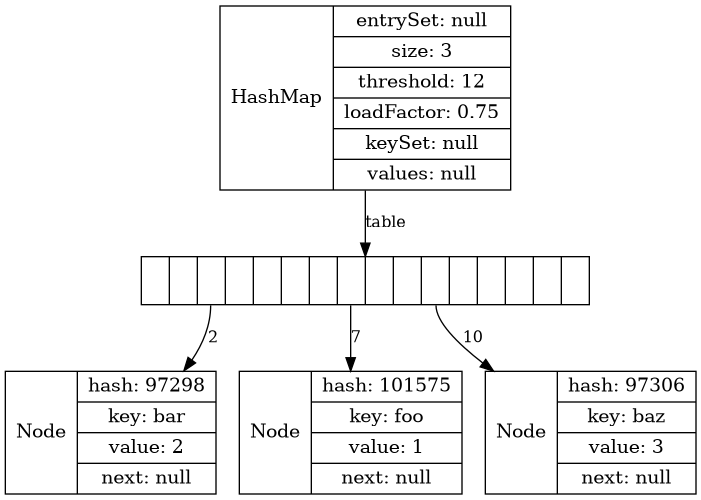## Hash collisions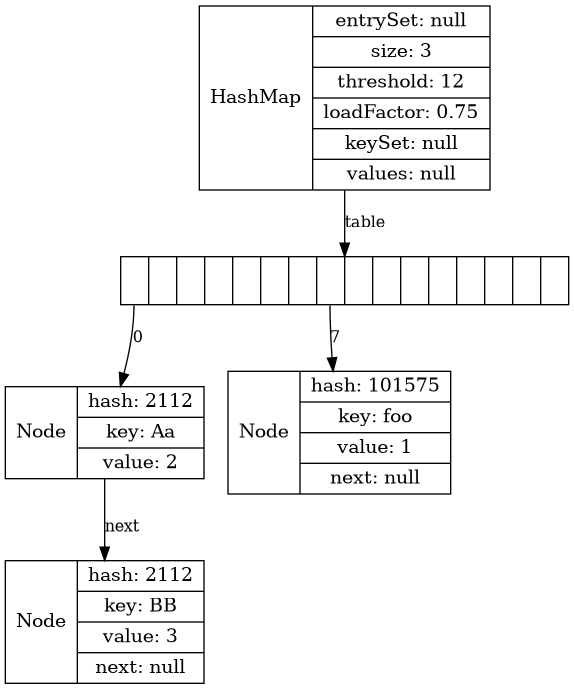``````Map map = new HashMap<>(); map.put("foo", 1); map.put("Aa", 2); map.put("BB", 3);`````` If there are a large number of collisions on one cell, if the value implements `Comparable`, the linked list is replaced with a tree.Implement `Comparable`!!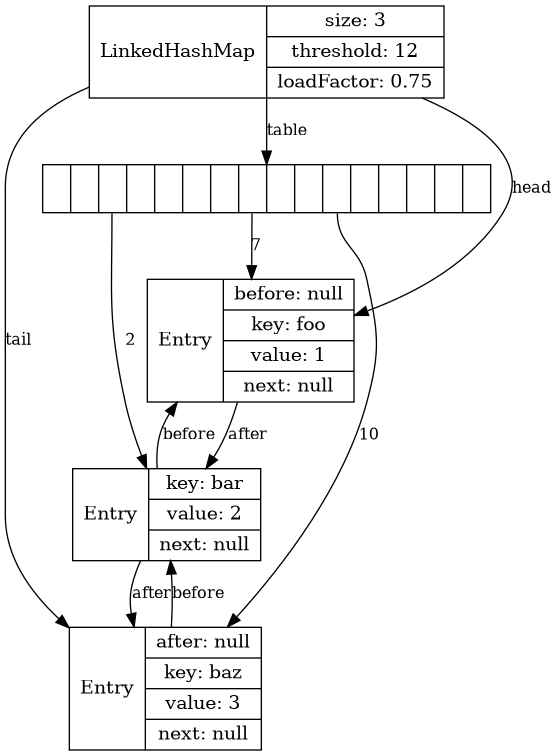``````Map map = new LinkedHashMap<>(); map.put("foo", 1); map.put("bar", 2); map.put("baz", 3);`````` Maintains element insertion order.Good for LRU cache.Good for keeping key-value settings (e. g. stored in `.properties` file).

## More variations on the hash table theme

• `IdentityHashMap` — keys are compared by `==` rather than `equals()`.

• `WeakHashMap` — values can be garbage collected if not used elsewhere.

## TreeMap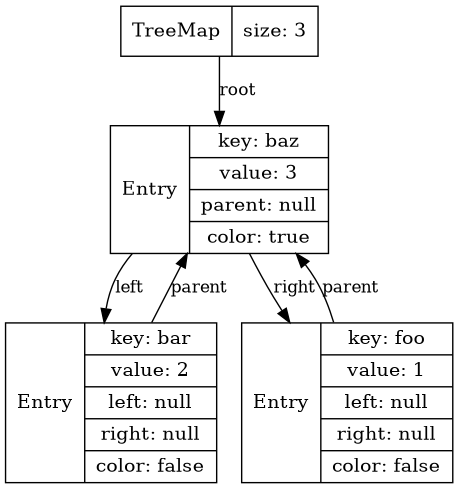``````Map map = new TreeMap<>(); map.put("foo", 1); map.put("bar", 2); map.put("baz", 3);`````` Red-black tree, keys are compared by `Comparable` or `Comparator`.If used as an ordinary `Map` is inferior to `HashMap`,Indispensable in cases where the key is known only approximately.

## Sets

• Collections in which an object can only be present once.

• Implemented on the basis of the corresponding Maps:

• `HashSet`,

• `LinkedHashSet`,

• `TreeSet`.

``````private transient HashMap<E,Object> map;
// Dummy value to associate with an Object in the backing Map
private static final Object PRESENT = new Object();

return map.put(e, PRESENT)==null;
}``````

## Bitmap-based sets

• `EnumSet` — uses a single `long` if there is less than 64 enum values. Use it for enums!

• `BitSet` — array of `long`s.

## Algorithms: sorting and shuffling

 ``````/*Implements Comparable*/ List names = ... Collections.sort(names); /*We pass Comparator*/ List staff = ... Collections.sort(staff, Comparator.comparingLong( Employee::getSalary)); /*Sometimes we need to shuffle*/ List cards = ... Collections.shuffle(cards);`````` ``````/*Implements Comparable*/ String[] names = ... Arrays.sort(names); /*We offer Comparator*/ Employee[] staff = ... Arrays.sort(staff, Comparator.comparingLong( Employee::getSalary)); /*There is no Arrays.shuffle!*/``````

• min/max search

• copying

• list reversing

• union and difference

• …​ — search and you will find!

## Callbacks: before lambdas

``````public interface ActionListener {
void actionPerformed(ActionEvent event);
}

// ----using----
String text = ...
new Timer(1000, new ActionListener(){
@Override
public void actionPerformed(ActionEvent e) {
... //'text' var is available here
}
});``````

## Callbacks: Comparator

``````String[] friends = {"Peter", "Paul", "Mary"};

Arrays.sort(friends,
new Comparator<String>() {
@Override
public int compare(String o1, String o2) {
return o1.length() - o2.length();
}
});``````

## Predicate

``````File[] hiddenFiles = new File(".").listFiles(
new FileFilter() {
public boolean accept(File file) {
return file.isHidden();
}
}
);``````

## Welcome lambda expressions!

``````//single-line
(String first, String second) ->
first.length() - second.length()

//multi-line with int return
(String first, String second) -> {
if (first.length() < second.length()) return -1;
else if (first.length() > second.length()) return 1;
else return 0;
}

//no-arg, void return
() -> System.out.println("Hello!");``````

## Which of these are valid lambda expressions?

1. `() → {}`

2. `() → "Raoul"`

3. `() → {return "Mario";}`

4. `(Integer i) → return "Alan" + i`

5. `(String s) → {"Iron Man"}`

Answer: 1-3. If lambda contains curly braces, be sure to use `return`. No curly braces — no `return` needed!

## What can lambdas be assigned to?

• A functional interface is one that has no more than one abstract method (it is clear what to run).

• Can be tagged with `@FunctionalInterface`, although it is not required.

• If the interface method matches the parameters and the return value of the lambda - then it can be assigned.

``````//You don't need to specify the types of lambda arguments: type inference!
ActionListener = e -> {...}
Comparator<String> c = (s1, s2) -> s1.length() - s2.length();``````

## Void-compatibility

Both options are compiled:

``````final List<String> list = ...

//Predicate.test returns boolean
Predicate<String> p = s -> list.add(s);

//Consumer.accept returns void!
Consumer<String> c = s -> list.add(s);``````

## `Object` is not a functional interface!

``````//will not compile
Object o = ()->{};

//Compiled!
Runnable r = ()->{};

//Also compiled
Object o = (Runnable) ()->{};``````

## As a result we have

 Anonymous Class Lambda ``````new Timer(100, new ActionListener(){ @Override public void actionPerformed(ActionEvent e) { ... } });`````` ``new Timer(100, e -> {...});`` ``````Arrays.sort(friends, new Comparator() { @Override public int compare( String o1, String o2) { return o1.length()-o2.length(); } });`````` ``````Arrays.sort(friends, (s1, s2)-> s1.length() - s2.length());``````

## Closures

``````void repeatMessage(String text, int delay) {
ActionListener  listener = event -> {
//text variable is accessible inside the lambda!
System.out.println(text);
}
new Timer(delay, listener).start();

}``````
• Lambda "ingridients":

• Code

• Parameters

• Captured variables that must be final or effectively final

## Effectively final only

``````int start = ...

for (int i = 0; i < count; i++) {
ActionListener linstener = event -> {
start--; //ERROR: Can't mutate captured value

//ERROR: Cannot refer to changing i
System.out.println(i);
}
}``````

(Effectively final variables are those that are either already `final`, or, if you put `final` for them, the code will still compile)

## Method references: even shorter, even more efficient

 ``event -> System.out.println(event)`` ``System.out::println`` ``(s1,s2)->s1.compareToIgnoreCase(s2)`` ``String::compareToIgnoreCase`` ``(x, y) -> Math.pow(x,y)`` ``Math::pow``

## Three ways to define a Method reference

 `object::method` `(x, y, z…​) → object.method(x, y, z…​)` `Class::instanceMethod` `(x, y, z…​) → x.instanceMethod(y, z…​)` `Class::staticMethod` `(x, y, z…​) → Class.staticMethod(x, y, z…​)`

## Constructor/Array Constructor Reference

 `Class::new` `(x, y, z…​) → new Class(x, y, z…​)` `Class[]::new` `(int i) → new Class[i]`

## IDE will help, but there is a difference!

``````obj = null;

//NPE on lambda invocation only!!
//obj must be effectively final!
doSmth(x -> obj.method(x));

//NPE here and now
//obj must not be effectively final
doSmth(obj::method)``````

## Methods specially created to be method references

``````//Come on, is it hard to check for null??
Objects.isNull(Object obj)...
Objects.nonNull(Object ob)...

list.removeIf(Objects::isNull);

stream.filter(Objects::nonNull)...``````

Functional InterfaceParameter TypesReturn TypeAbstract Method NameDefault Methods

Runnable

none

void

run

Supplier<T>

none

T

get

Consumer<T>

T

void

accept

andThen

BiConsumer<T, U>

T, U

void

accept

andThen

## Combination of consumers

``````Consumer<String> foo = ...
List<String> list = ...

//Compound consumer sending
//object first in the first, then in the second

## Functions

Functional InterfaceParameter TypesReturn TypeAbstract Method NameDefault Methods

Function<T, R>

T

R

apply

compose, andThen, identity

BiFunction<T, U, R>

T, U

R

apply

andThen

## Composition of functions and identity

 `f.andThen(g)` `g(f(x))` `f.compose(g)` `f(g(x))` `Function.identity()` `x → x`

Why does `BiFunction` have no `compose`, only `andThen`?

## Operators

Functional InterfaceParameter TypesReturn TypeAbstract Method NameDefault Methods

UnaryOperator<T>

T

T

apply

compose, andThen, identity

BinaryOperator<T>

T, T

T

apply

andThen, maxBy, minBy

## Composition of operators

• `UnaryOperator<T> extends Function<T,T>`, that’s why `compose`, `andThen` and `identity` work the same way.

• `BinaryOperator<T> extends BiFunction<T,T,T>`, that’s why `andThen` works the same way

• The static `minBy` and `maxBy` methods generate the `min(x,y)` and `max(x,y)` operators from the comparator.

## Predicates

Functional InterfaceParameter TypesReturn TypeAbstract Method NameDefault Methods

Predicate<T>

T

boolean

test

and, or, negate, isEqual

BiPredicate<T, U>

T, U

boolean

test

and, or, negate

## Predicate composition

``````Predicate<T> a = ...
Predicate<T> b = ...

a.and(b).negate(); // ! (a(x) & b(x)

//Double predicate (x,y)-> Objects.equals(x, y)
Objects::equals

//Single predicate y -> Objects.equals(x, y)
Predicate.isEqual(x)``````

## Functional interfaces for primitive types

p, q is `int`, `long`, `double`; P, Q is `Int`, `Long`, `Double`

Functional InterfaceParameter TypesReturn TypeAbstract Method Name

BooleanSupplier

none

boolean

getAsBoolean

PSupplier

none

p

getAsP

PConsumer

p

void

accept

ObjPConsumer<T>

T, p

void

accept

PFunction<T>

p

T

apply

PToQFunction

p

q

applyAsQ

ToPFunction<T>

T

p

applyAsP

ToPBiFunction<T, U>

T, U

p

applyAsP

## Functional interfaces for primitive types (continued)

Functional InterfaceParameter TypesReturn TypeAbstract Method Name

PUnaryOperator

p

p

applyAsP

PBinaryOperator

p, p

p

applyAsP

PPredicate

p

boolean

test

## Default `Map` interface methods

Work atomically in `ConcurrentHashMap`!

``````V computeIfAbsent(K key,
Function<? super K, ? extends V> mappingFunction)

V computeIfPresent(K key,
BiFunction<? super K, ? super V, ? extends V> remappingFunction)

V compute(K key,
BiFunction<? super K, ? super V, ? extends V> remappingFunction)

V merge(K key, V value,
BiFunction<? super V, ? super V, ? extends V> remappingFunction)``````

## Comparators

``````@AllArgsConstructor
public class Person {
@Getter
private final String firstName;
@Getter
private final String lastName;
}
List<Person> people = new ArrayList<>();``````

## Sort by last name

 ``````/*WRONG*/ Collections.sort(people, (p1, p2) -> p1.getLastName() .compareTo( p2.getLastName()); );`````` ``````/*CORRECT*/ Collections.sort(people, Comparator.comparing (Person::getLastName)); );``````

## Sort by last name, then by first name

``````//DON'T WRITE THIS HORRIBLE MESS
Collections.sort(people,
(p1, p2) -> {
int result = p1.getFirstName().compareTo(p2.getFirstName());
if (result == 0) {
result = p2.getLastName().compareTo(p2.getLastName());
}
return result;
}
);``````

## Correct:

``````Collections.sort(people,
Comparator
.comparing(Person::getLastName)
.thenComparing(Person::getFirstName));
);``````

## Also:

``````Comparator.comparing(keyExtractor, keyComparator)
Comparator.comparingInt/Double(...)
Comparator.reversed()
Comparator.nullsFirst/nullsLast(Comparator c)``````